• 6-75. See below:
1. 26
2. 29
3. 9
• 6-91. See below:
1. A = 12 un.2, P = 14 un.
2. A = 10 un.2, P = 14 un.
3. Answers vary, but should simplify to A = 3x un.2, P = 2x + 6 un.
4. The last shape uses algebra tiles, so it is not possible to describe the “toothpicks” or “tiles” as a single number.
5. Because   can represent different lengths, you cannot tell if the perimeter is greater or less than the perimeter of shape (a).
• 6-96. See below:
1. 4+ 4 units
2. 4x + 4 units
• 6-106. See below:
1. P = 4+ 4 units, A = x2 + x + 1 square units
2. P = 2x + 4 units, A = x + 1 square units
3. P = 4x + 6 units, A = x2 + 2x + 1 square units
• 6-107.  The picture should contain 5 x2‑tiles, 8 x‑tiles, and 3 unit tiles.  A = 5x2 + 8x + 3 square units
• 6-110. See below:
1. 21
2. 14
3. 22
• 6-115. See below:
1. 32 units
2. 26 units
3. 13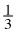units
• CL 6-121.

a.  P = 4x + 6 units
b.  A = x2 + 2x + 2 square units
c.  The area would not change.

• CL 6-122.

a.  5                                   b.  16
c.  25                                 d.  4

• CL 6-126.

a. x2 + 7x + 2              b. 18x + 3
c. 3x2 + 3x + 8            d. 4x + 6

• 7-9. See below:
1. \$144 ÷ \$36 = 4 sets of 4 hours, 16 hours
2.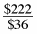≈ 6.167 sets of 4 hours,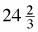hours
3. \$360 for a 40-hour week, \$63 for a 7 hour day.
• 7-19. See below:
1. See sample graph below.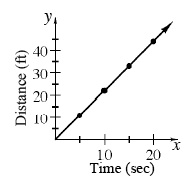2.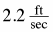3. Steeper, because she is traveling faster at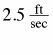.
• 7-30. See below:
•      DWest, because it has a unit rate of \$0.15/min.
• 7-31. See below:
1. C
2. \$4 per pound
3. Store A. Its line is steepest.
• 7-40. See below:
1.
• 7-60. See below:
1.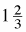2. 10
3.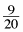• 7-64. See below:
1.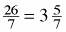2. 3.25
3.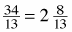• 7-74.  See below:
1.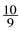= 1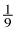2. 10
3.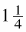• 7-75. 12
• CL 7-131.

Eva is saving at a faster rate, because she saves \$75 a week, while Annie saves about \$72 a week.

• CL 7-133.

Values in the table are:
(6 cans, 10 dollars), (9 cans, 15 dollars), (27 cans, 45 dollars), (30 cans, 50 dollars)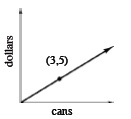• CL 7-135.

6 ÷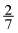= 21  cupcakes

All answers taken from CPM ebook CC1